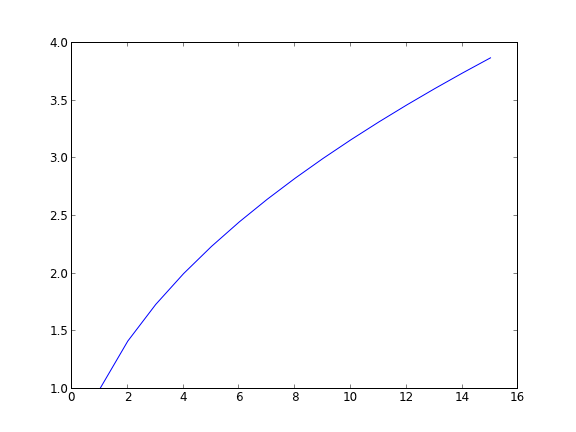Interacting with Excel in python

| categories: programming | tags: | View Comments

There will be times it is convenient to either read data from Excel, or write data to Excel. This is possible in python (http://www.python-excel.org/). You may also look at (https://bitbucket.org/ericgazoni/openpyxl/wiki/Home).

import xlrd

wb = xlrd.open_workbook('data/example.xlsx')
sh1 = wb.sheet_by_name(u'Sheet1')

print sh1.col_values(0)  # column 0
print sh1.col_values(1)  # column 1

sh2 = wb.sheet_by_name(u'Sheet2')

x = sh2.col_values(0)  # column 0
y = sh2.col_values(1)  # column 1

import matplotlib.pyplot as plt
plt.plot(x, y)
plt.savefig('images/excel-1.png')

[u'value', u'function']
[2.0, 3.0]1 Writing Excel workbooks

Writing data to Excel sheets is pretty easy. Note, however, that this overwrites the worksheet if it already exists.

import xlwt
import numpy as np

x = np.linspace(0, 2)
y = np.sqrt(x)

# save the data
book = xlwt.Workbook()

for i in range(len(x)):
sheet1.write(i, 0, x[i])
sheet1.write(i, 1, y[i])

book.save('data/example2.xls') # maybe can only write .xls format


2 Updating an existing Excel workbook

It turns out you have to make a copy of an existing workbook, modify the copy and then write out the results using the xlwt module.

from xlrd import open_workbook

from xlutils.copy import copy

rb = open_workbook('data/example2.xls',formatting_info=True)
rs = rb.sheet_by_index(0)

wb = copy(rb)

ws.write(0, 0, "Appended")

wb.save('data/example2.xls')


3 Summary

Matlab has better support for interacting with Excel than python does right now. You could get better Excel interaction via COM, but that is Windows specific, and requires you to have Excel installed on your computer. If you only need to read or write data, then xlrd/xlwt or the openpyxl modules will server you well.